# 【概述】

Dijkstra 算法是单源最短路径算法，即计算起点只有一个的情况到其他点的最短路径，其无法处理存在负边权的情况。

# 【算法分析】

Dijkstra 算法的思想，就是一开始将起点到终点的距离标记为 0，而后进行 n 次循环，每次找出一个到起点距离 dis[u] 最短的点 u ，将它从蓝点变为白点，随后枚举所有白点 Vi，如果以此白点为中转到达蓝点 Vi 的路径 dis[u]+w[u][vi] 更短的话，这将它作为 Vi 的更短路径（此时还不能确定是不是Vi的最短路径）。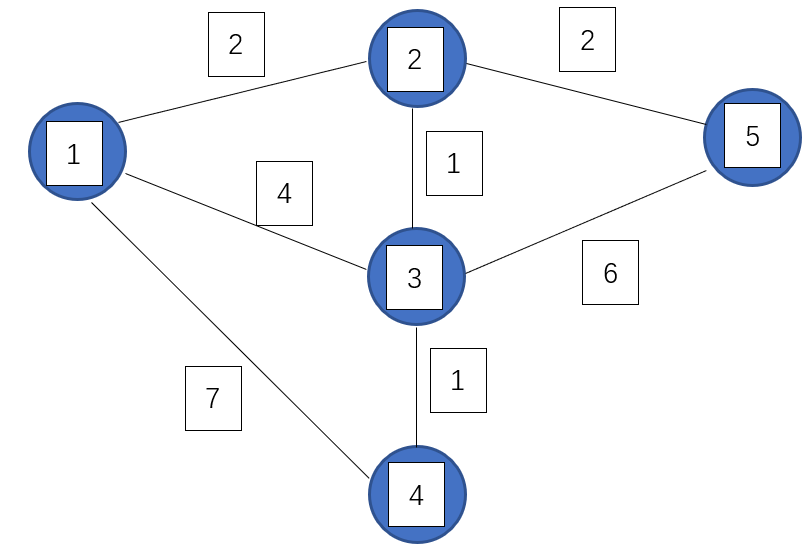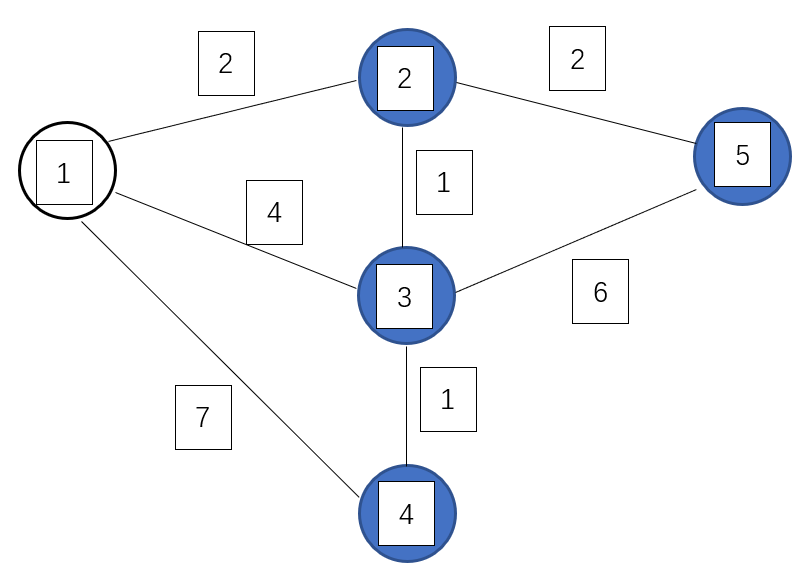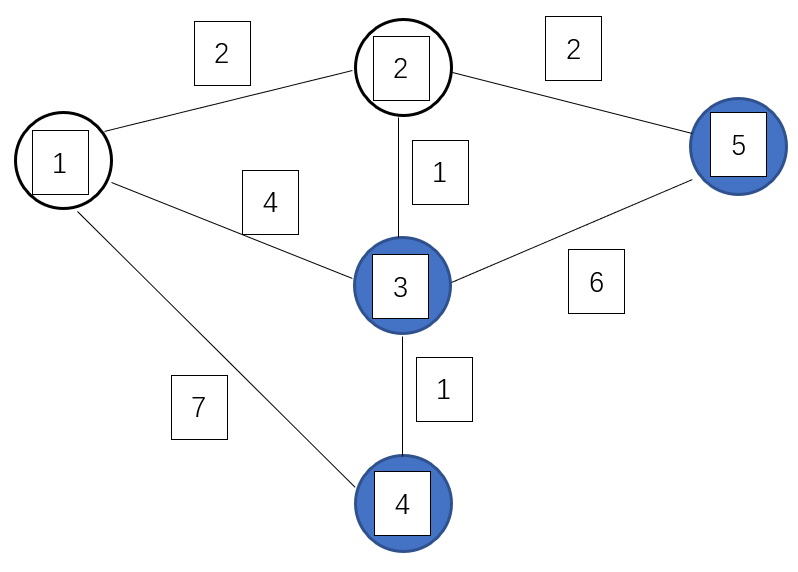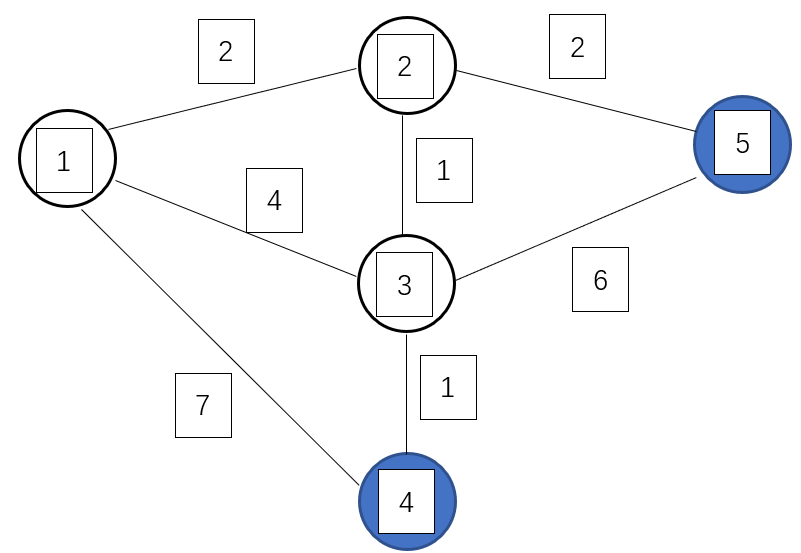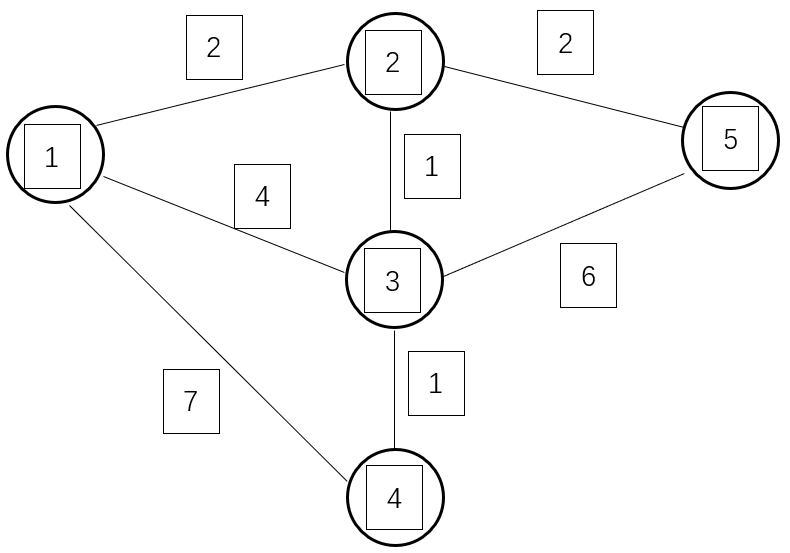N轮循环结束，所有点的最短路径均可求出。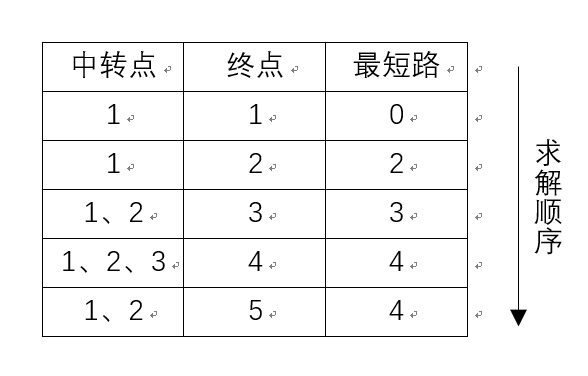# 【算法描述】

1.初始化：

dis[v]=0x3f3f3f3f（v≠s），vis[v]=false，即：从始点到各点的值初始化为一极大值，所有点均标记为未访问

dis[s]=0，pre[s]=0，即：始点到始点的距离为 0，且其没有前驱结点

2.算法主体：

``````for(int i=1;i<=n;i++) {
int min=INF;
int u=0;

for(int v=1;v<=n;v++) { //在没有被访问过的点中找一个顶点u，使得dis[u]是最小的
if( vis[v]==false && dis[v]<min) {
min=dis[v];
u=v;
}
}
if(u==0)
break;

vis[u]=true;//u标记为已确定的最短路径
for(int v=1;j<=n;j++) { //枚举与u相连的每个未确定的最短路的顶点
if(dis[u]+w[u][v]<dis[v]) {
dis[j]=dis[u]+w[u][v]; //更新最短路径
pre[v]=u;//记录前驱
}
}
}``````

3.算法结束：

dis[v] 即为 s 到 v 最短距离，pre[v] 即为 v 的前驱结点，用来输出路径。

# 【模版】

## 1.简化版

``````int dis[N];//单源最短距离
int G[N][N];//G[i][j]表示i到j的有向边长
bool vis[N];//表示w[i]是否已经计算完
void dijkstra(int n,int s){
for(int i=1;i<=n;i++){
int x;//x标记当前最短w的点
int min_dis=INF;//记录当前最小距离

for(int y=1;y<=n;y++){
if(!vis[y] && min_dis>=dis[y]){
x=y;
min_dis=dis[x];
}
}

vis[x]=true;

for(int y=1;y<=n;y++)
dis[y]=min(dis[y],dis[x]+G[x][y]);
}
}
int main(){
memset(dis,INF,sizeof(dis));
memset(vis,0,sizeof(vis));

int n,m;
scanf("%d%d",&n,&m);
for(int i=1;i<=m;i++){
int x,y,dis;
scanf("%d%d%d",&x,&y,&dis);
G[x][y] = G[y][x] = dis; //无向图添边一次,有向图添边两次
}
int start;
scanf("%d",&start);
dijkstra(n,start);
for(int i=1;i<=n;i++)
printf("%d\n",dis[i]);
return 0;
}``````

## 2.标准版

``````int n,m;
struct Edge{//边
int from;//边的起点
int to;//边的终点
int dis;//边的长度
Edge(int f,int t,int d){//构造函数
from=f;
to=t;
dis=d;
}
};

struct HeapNode{//Dijkstra用到的优先队列的结点
int dis;//点到起点距离
int u;//点的序号
HeapNode(int a,int b){
dis=a;
u=b;
}
bool operator < (const HeapNode &rhs) const  {
return dis > rhs.dis;
}
};

struct Dijkstra{
int n,m;//点数、边数
vector<Edge> edges;//边列表
vector<int> G[N];//每个结点出发的边的编号
bool vis[N];//是否走过
int dis[N];//起点s到各点的距离
int p[N];//从起点s到i的最短路中的最后一条边的编号

void init(int n) {//初始化
this->n = n;
for(int i=0;i<n;i++) //清空邻接表
G[i].clear();
edges.clear();//清空边列表
}

void AddEdge(int from,int to,int diss) {//添加边，若为无向图，调用两次
edges.push_back(Edge(from,to,diss));
m=edges.size();//边的个数
G[from].push_back(m-1);//添加至边列表
}

int dijkstra(int s) {//求s到所有点的距离

memset(dis,INF,sizeof(dis));
memset(vis,false,sizeof(vis));
dis[s]=0;

priority_queue<HeapNode> Q;//优先队列
Q.push(HeapNode(0,s));
while(!Q.empty()) {
HeapNode x=Q.top();
Q.pop();

int u=x.u;
if(vis[u])//若已被访问
continue;

vis[u]=true;//标记为已访问
for(int i=0;i<G[u].size();i++) {//枚举所有与当前点相连的边
Edge &e=edges[G[u][i]];
if(dis[e.to] > dis[u]+e.dis) {//更新距离
dis[e.to] = dis[u]+e.dis;
p[e.to]=G[u][i];
Q.push(HeapNode(dis[e.to],e.to));
}
}
}
return dis[n];//返回起点s到终点n最短距离
}
}DJ;

int main()
{
while(scanf("%d%d",&n,&m)!=EOF&&(n+m))
{
DJ.init(n);//初始化
for(int i=0;i<m;i++) {
int x,y,dis;
scanf("%d%d%d",&x,&y,&dis);
//无向图添边两次
}

int start;
scanf("%d",&start);
DJ.dijkstra(start);//求start到各点的距离
for(int i=0,j=0,s=++start;i<n;i++)//输出start到各点的距离
printf("%d->%d: %d\n",s,++j,DJ.dis[i]);
}
return 0;
}``````

### Spring @Configurable基本用法_weixin_34099526的博客-程序员宝宝

2019独角兽企业重金招聘Python工程师标准&gt;&gt;&gt; ...

### Python3.5源码分析-内建模块builtins初始化_小屋子大侠的博客-程序员宝宝

Python3源码分析本文环境python3.5.2。参考书籍&amp;amp;lt;&amp;amp;lt;Python源码剖析&amp;amp;gt;&amp;amp;gt;python官网Python3模块初始化与加载Python的模块分为内建的模块，函数与用户定义的模块，首先分析Python内建模块。Python3的系统内建模块初始化上文介绍了Python的线程对象和解释器对象，在初始化的时候，会执行_Py_Initi...

### Jetpack Hilt 依赖注入框架上手指南_weixin_38754349的博客-程序员宝宝

code小生 一个专注大前端领域的技术平台公众号回复Android加入安卓技术群作者：LvKang-insist链接：https://juejin.im/post/5efdff9d6fb...

ffmpeg

### json c语言开发,Parson - 用C语言编写的轻量级JSON库_WxZz呀的博客-程序员宝宝

AboutParson is a lighweight json library written in C.FeaturesFull JSON supportLightweight (only 2 files)Simple APIAddressing json values with dot notation (similar to C structs or objects in most OO ...

### Hyperledger Fabric2.0.0报错集锦_Yesterjunior的博客-程序员宝宝

Hyperledger Fabric报错集锦文章目录Hyperledger Fabric报错集锦(一)安装配置过程中1. go get 无法下载(一)安装配置过程中1. go get 无法下载当我在test-network目录下执行./network.sh deployCC 命令时:Error: failed to normalize chaincode path: 'go list' ...

### Spring Security基础-1-HttpBasic基本认证登录_.httpbasic 改成自定义_springboot葵花宝典的博客-程序员宝宝

Spring Security基础-1-HttpBasic基本认证登录Spring Security简介 Spring Security的前身是AcegiSecurity，收入到Spring子项目以后改名为Spring Security。Spring Security的核心功能有两个认证和授权Authentication：**就是身份认证(简称认证)**，用来告诉系统你是谁的Authorization：**就是访问授权(简称授权)**，当系统指定你是谁以后,就会根据权限管理获取你应有的权限，告诉你可以干什么